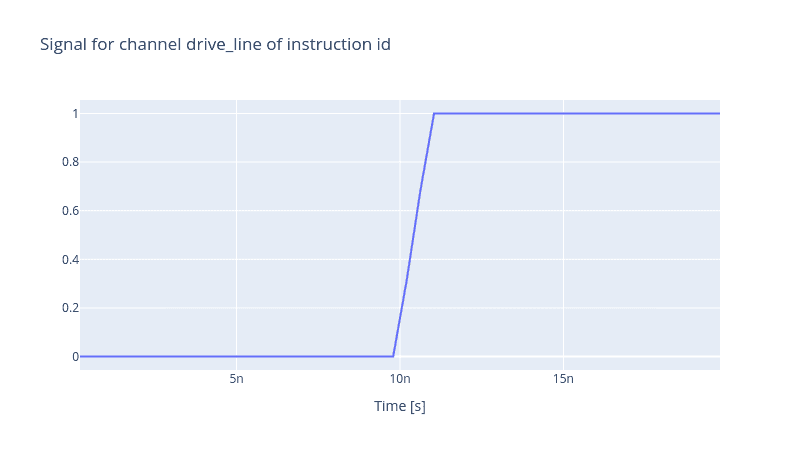# Realistic Control Stack Modelling: Part III - Rise Time

### Rise Time

Electronic devices do not respond instantaneously to changes when the input signal alters. For instance, when you switch on a light bulb, it takes a while for the bulb to achieve the highest luminescence. This delay is called rise time. Although it is desirable to have a long rise time, for instance, in the case of the light bulb to increase its lifetime, it is crucial to reduce this delay in high-speed electronics. Specifically, in quantum control problems, long delays result in distorted pulses that affect the fidelity of gate instruction.

To model such a finite delay of the electronic devices in the control stack, in the Qruise toolset, we have implemented a pseudo-device called `RiseTime` that mimics and simulates the delay specific to each electronic device. In their specification sheets, rise time characterises the time it takes for the output of a step function signal to reach from 20% to 80% of the maximum amplitude value, which is quite a standard range but can indeed vary. In the Qruise toolset, such behaviour is modelled by convoluting the pulse with a Gaussian filter. The difference between 80% and 20%, i.e. 0.6, defines the width of the Gaussian filter.

In this blog post, we will investigate the effect of rise time in a control stack constituting a single AWG device. Like always, let us define our flux line.

``````from qruise.toolset.libraries import components, hamiltonians

drive_line = components.Drive(
name="flux_line",
connected=["Qubit_0"],
hamiltonian_func=hamiltonians.x_drive,
)
``````

The RiseTime device receives two parameters. The first one is `fraction` which specifies the difference between the minimum and maximum amplitude percentages i.e. the value 0.6 implies the difference between 80% and 20% of the maximum amplitude. And the second parameter is `rise_time` which specifies how long it takes for a pulse to reach from the minimum amplitude fraction to the maximum amplitude fraction. The code block below shows how this is achievable in the Qrusie toolset.

``````from qruise.toolset.objects import Quantity as Qt
from qruise.toolset.generator.devices import OEMAWG, RiseTime, VoltsToHertz

# simulation resolution, different than the AWG sampling rate
sim_res = 1e11

awg_params = {
"name": "AWG",  # given name of the device
"sim_time": 20e-9,  # 20ns (pulse duration)
"samp_rate": int(2.4e9),  # 2.4 GHz sample rate
"waveform_granularity": 8,  # granularity of the AWG device
"min_waveform_len": 16,  # shortest (in number of samples) waveform AWG can produce
}

risetime_devece_params = {
"rise_time": Qt(value=450e-12, unit="s"),
"fraction": Qt(value=0.6, unit=""),
}

stack_devices = {
"AWG": OEMAWG(**awg_params),
"RiseTime": RiseTime(name="RiseTime", **risetime_devece_params),
"V2Hz": VoltsToHertz(
name="V2Hz", V_to_Hz=Qt(value=1.0, unit="V/Hz")
),
}

stack_chain = {"drive_line": {"AWG": [], "RiseTime": ["AWG"], "V2Hz": ["RiseTime"]}}
``````

The rise time is actually measured for a step function. Hence, it is natural to define the envelope of the pulse generated by the AWG to be a rectangular pulse.

``````from qruise.toolset.signal import pulse

t_gate = stack_devices["AWG"].sim_time

rect_params = {
"amp": Qt(value=1.0, unit="V"),
"t_start": Qt(value=0.0, unit="s"),
"t_final": Qt(value=t_gate, unit="s"),
"delta": Qt(value=0, unit=""),
}

rect_env = pulse.Envelope(
name="rectangular",
params=rect_params,
shape=pulse.envelopes.rect,
)
``````

But the rectangular pulse remains constant for the whole simulation since the gate time `t_gate` and the simulation time `sim_time` are equal. To generate a step-function envelope, we can add a delay to the envelope and only start generating it when we are half-way through the simulation time. This `delay` option is passed to the `Instruction` when we want to add the envelope to it. We create an identity instruction and assert that the envelope should only be generated for time stamps larger than `t_gate / 2`.

``````from qruise.toolset.signal import gates
from qruise.toolset.libraries import constants

instr = gates.Instruction(
name="id",  # name of the instruction
t_end=t_gate,  # duration of the instruction (pulse duration)
channels=["drive_line"],  # to which channel the instruction (pulse) is applied
targets=,  # the index of the target qubit (not relevant but mandatory)
ideal=constants.Id,
)

comp=rect_env, chan="drive_line", options={"delay": Qt(value=t_gate / 2)}
)
``````

Then instantiate the `Generator` and produce the output pulse.

``````from qruise.toolset.generator.generator import Generator

generator = Generator(devices=stack_devices, chains=stack_chain)
generator.draw(instr=instr, resolution=stack_devices["AWG"].samp_rate)
``````Actually, it is possible to measure the rise time by finding the indices of the 20% and 80% of the maximum amplitude. Once found, we extract the timestamps associated with these indices and subtract them consequently.

``````import numpy as np

signal = generator.generate_signals(
instr=instr, resolution=8 * stack_devices["AWG"].samp_rate
)  # get the signal of the whole stack with a higher resolution compared to the AWG sample late

# get the timestamps
ts = signal.signal["drive_line"]["ts"]
# get the pulse generated by the RiseTime device
output_pulse = signal.signal["drive_line"]["signal_stack"]["RiseTime"]["inphase"]
# get the index of 20% of the maximum value
ind_2 = np.where(output_pulse.numpy() < 0.2).max()
# get the index of 80% of the maximum value
ind_8 = np.where(output_pulse.numpy() > 0.8).min()

risetime = (ts[ind_8] - ts[ind_2]).numpy() / 1e-12
print(f"Rise time of {risetime: .2f}ps")
``````
``````Rise time of  468.75ps
``````

The value is slightly different from the actual value 450ps we set earlier when we defined the `RiseTime` object, but this is due to the discretisation of time when we set the resolution of the `Generator`. The finer the simulation resolution is, the more precise the resulting rise time will be.

Back to all posts